## Electric Field - Complete Toolkit

### Objectives

1. To understand that all sources of charge create an influence or action upon other objects some distance away and that the electric field concept is used to describe that influence.
2. To state the mathematical definition of electric field (force/charge) and to describe the dependence of the electric field strength upon the variables that affect it.
3. To use an understanding of the convention for electric field direction to identify the electric field direction around a source charge.
4. To construct and to interpret electric field line diagrams for isolated charges and for collections of two or more charges.
5. To use the electric field equation, Coulomb’s law equation, and Newton’s laws to analyze physical situations that involve electric fields and to solve physics word problems associated with such situations.

### Interactive Simulations

1. The Physics Classroom Interactive: Electric Field Lines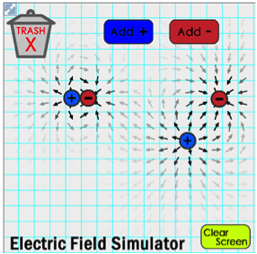Beginners can get a feel for electric field patterns with this simple simulation appropriate for Conceptual Physics or Physics First courses. Learners drag positive or negative charges onto the field and observe the field lines that result from the configuration of charges. Note: Specifically meets NGSS High School DCI: HS-PS2.B.2 – "Forces at a distance are explained by fields permeating space that can transfer energy through space."

2. The Physics Classroom Interactive: Electrostatics Landscapes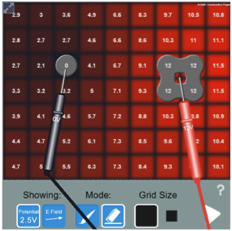This simulation was designed to help learners visualize the electric potential surrounding two point charge sources. Place two charges anywhere along the virtual conductive paper, tap Start, and observe the system approach equilibrium. You can view the landscape in two ways: 1) as electric field lines surrounding the charges, or 2) as electric potential shown in volts.

3. The Physics Classroom Interactive: Put the Charge in the Goal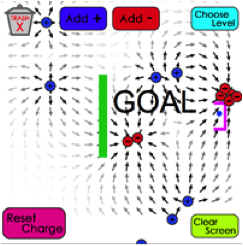Have Phun with the Physics by using your understanding of electric fields to guide a charged puck around obstacles to a goal. By placing charges on the virtual rink, your puck can be attracted or repelled around obstacles. Each time you level up, additional barriers are added!

4. PhET Simulation:  Charges and FieldsWhat variables affect how charged bodies interact? In this model, students drag a positive or negative charge (or both) onto the field, then place “E-field sensors” (small positive test charges). Tools include a virtual tape measure and a voltage sensor. Arrows show the force vectors. Registered PhET users can access a set of teacher-contributed lessons and student guides.

5. Point Charge Electric Field and Potential in 2D ModelFor the AP physics class, this Java model explores electric field and potential in a 2-dimensional situation. Students can turn on 1-5 charged particles, then move a test charge around the plane to sample both the electric field and potential. You can turn on a grid of field vectors that show the direction. Net electric potential and magnitude of the net field are shown numerically in a table. The 3 key takeaways:  1) If the charge is positive the direction of the electric field is away from the charge, if negative the direction is toward the charge; 2) Electric field is a vector; and 3) Net electric field at any point is the vector sum of the fields due to individual charges.

### Video and Animations

1. Education Commons RW: Electric Fields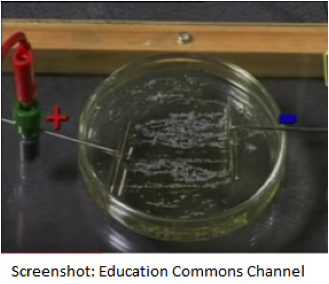This high-quality 9-minute video uses a combination of grass seed demonstrations and whiteboard illustrations to show patterns and direction of electric field lines. It promotes understanding of three big ideas: 1) Electric field patterns are predictable and can be mapped on a diagram, 2) Electric force is strongest near the charged object where field lines are closest together, and 2) Electric field lines and magnetic flux lines have similarities that can be helpful in understanding field properties of both. Great resource for students with reading disabilities or who are struggling with the content.

2. Veritasium: What’s in a Candle FlameWe know that fire is an oxidizing chemical reaction that releases heat and light. But is a flame plasma? One test case would be whether a candle flame becomes conductive when exposed to an electric field of sufficient magnitude. Physics education researcher and Veritasium host Derek Muller travels to Paris where he and a French physicist test this very idea. Editor’s Note: Scientists are divided in their views about whether flame is a plasma. We’re not taking a position here, but hope this video will “spark” a lively discussion!

3. AAPT Films: Electric Field Visualized with Crystals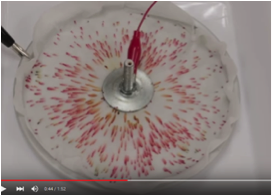Physics educator James Lincoln demonstrates electric field by using potassium permanganate crystals, an inorganic compound commonly used in water treatment plants. The crystals are allowed to dissolve around a positive electrode. The permanganate ion is negative, so it flows toward the electrode as it dissolves, creating a nice field pattern. Not recommended for live set-up in high school labs due to high voltage levels and toxicity of the chemical.

### Physics Education Research

1. Contrasting Students’ Understanding of Electric Field and Electric Force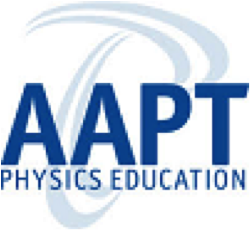This 2012 study compared to two sequences of student questions to gauge understanding of electric field. In one sequence, electric force representations were used and in the other, the electric field sequence. The study encompassed a field of 249 students at a large private Mexican university, all of whom had completed introductory courses in Mechanics and Electricity and Magnetism. Group 1 (n = 122) addressed the force sequence; group 2 (n = 129) took the electric field sequence. The key findings were: 1) Students have a much harder time understanding the electric field concept than the electric force concept; 2) Students answering correctly used mostly vector-based models; 3) Most students used field lines to represent electric field, rather than treating them as vectors, and 4) Even after a course in Electricity & Magnetism, most students did not understand the basic concepts underlying electric field lines.

### Labs and Investigations

1. The Physics Classroom, The Laboratory, Electric Field Simulation

Students use an Open Source Physics electric field simulation to explore the effect of distance, test charge amount, and source charge amount upon the strength of the electric field.

2. The Physics Classroom, The Laboratory, Coulomb’s Hair Lab

Charged balloons are hung by light strings from a common support. Students make measurements (mass, separation distance, string length, etc.) in order to determine the number of electrons transferred to two balloons when rubbed against animal fur.

3. The Physics Classroom, The Laboratory, Electric Field Lines

Students use an electric field line simulator to explore the pattern of field lines (direction, general shape, density, etc.) around single point charges and a collection of point charges.

### Demonstration Ideas

1. AAPT Films: Ten Ways to “See” the Electric FieldWe can’t see an electric field. So how can students visualize one? HS physics teacher and popular video host James Lincoln demonstrates how with his usual brand of humor and great science. The video explores ten experiments that could be done in the high school classroom. Editor’s Note: We especially like the Plasma Globe experiment, a great choice for the budget-conscious teacher (available at science supply stores for about \$50).

2. Equipotential Lines, Electric and Magnetic Field MappingThis “how-to” video gives great guidance in setting up a classroom lab on field mapping. It gives detailed directions for two methods that both employ conducting paper and voltmeter. In one method (shown above at right) conducting bars are used to produce the pattern. The second method produces a dipole pattern. The host gives tips on getting the best readings and shows you how to plot equipotential lines as well.

3. Rutgers University: Electric Potential and Field Maps Lab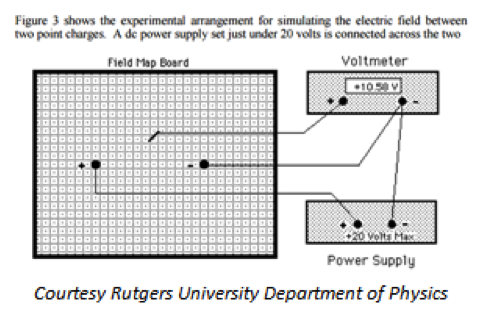This is a student guide for a lab on field mapping using a voltmeter and field map board. It explains procedures for doing the mapping with the apparatus and calculating electric potential. Appropriate for calculus-based AP Physics.

4. Physlet Physics:  Electric Field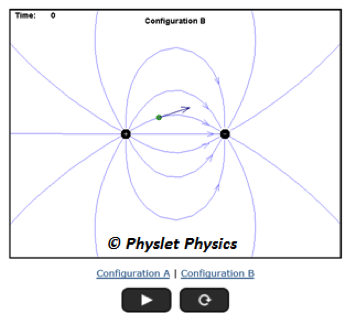If you haven’t tried Physlets for teaching introductory physics, you’re in for a treat! Physlets are simple Java models designed to deal with only one facet of a physical phenomenon and do not introduce much data analysis. The Electric Field Physlet package features four explanatory models for concept introduction; three interactive explorations with supplementary worksheets; and 10 animation-based problems. Sub-topics include field line representation, electric fields from point charges, fields and test charges, ranking tasks, and how to calculate the electric field.

### Minds On Physics Internet Modules:

The Minds On Physics Internet Modules are a collection of interactive questioning modules that target a student’s conceptual understanding. Each question is accompanied by detailed help that addresses the various components of the question.
1. Static Electricity, Ass’t SE10 - Electric Field

2. Static Electricity, Ass’t SE11 - Electric Field Equation

3. Static Electricity, Ass’t SE12 – Electric Field Lines

### Concept Building Exercises:

1. The Curriculum Corner, Static Electricity, Electric Field

2. The Curriculum Corner, Static Electricity, Electric Field Lines

### Problem-Solving Exercises:

1. The Calculator Pad, Static Electricity, Problems #16 - #21, #26 - #32

### Science Reasoning Activities:

1. Science Reasoning Center, Electrostatics, Charge Interactions

2. Science Reasoning Center, Electrostatics, Sticky Tape Experiments

### Common Misconception:

1. Field Strength is NOT Force Strength

It is important that students distinguish between electric force and electric field strength. They are not the same. They have different (albeit related) definitions, are expressed in different units, and involve a different set of measurements in order to determine them. The electric force describes the amount of push or pull exerted on an object by another charged object. The electric field strength describes the amount of force per unit of charge experienced by a test charge if placed at any given location about a source charge. Electric force has the standard unit of Newton (N) an electric field has the standard unit of Newton/Coulomb (N/C).  (See Related Physics Education Research on Page 1 for more insight into this persistent misconception.)

2. Convention for Direction

The direction of the electric field around a source charge is not always the same as the direction of the force upon a test charge. By convention, the electric field direction is the direction that a positive test charge would be pushed or pulled if placed in a given space. As such, the direction of force on a negative charge would be in a direction that is opposite of the electric field direction.

3. Electric Field Lines Show the Direction of Force

It is a common student misconception that the field lines surrounding a charged object show which direction a test charge would move if located on that line. They don’t. The field lines are lines of force, not lines of movement. Being lines of force, they only show the direction of force upon a charge object when momentarily located upon the line. As such, a test charge will not necessarily follow a motion directed along the line. For instance, if a moving charge approaches a line with a velocity that is directed at some angle to the line, the force will cause the test charge to deviate from its original path. However, it will not cause the test charge to continue in motion along the line.

### Elsewhere on the Web:

1. Point Charge Electric Field Demo ModelThis package from Open Source Physics offers a powerful modeling-based lesson for the latter phase of a unit on electric field. The simulator lets students explore electric field with multiple point charge configurations and vector field representations. It was designed to address the misconception that electric field and electric force are the same thing, and to promote understanding of factors affecting electric field strength. The package includes full lesson plan with warm-ups, a comprehensive assessment (with answer key), and modifiable jar file.

2. Spiral Physics: The Electric Field – Concepts and PrinciplesThis resource is part of Spiral Physics, a research-based curriculum for calculus-based physics courses. It features alternative problem types, goal-less problem statements, ranking tasks, and critical analysis. Topics are arranged to provide repeated exposure to concepts over time, with incremental step-up in complexity. This link goes directly to the chapter on Electric Field. It includes sections on analysis tools, point charges, continuous charge and distribution, Gauss’ Law, and charge density.

### Standards:

A. Next Generation Science Standards (NGSS)

Disciplinary Core Ideas: Types of Interactions
• HS-PS2.B.1:  Coulomb’s Law provides the mathematical model to describe and predict the effects of electrostatic forces between distant objects.
• HS-PS2.B.2:  Forces at a distance are explained by fields (gravitational, electric, and magnetic) permeating space that can transfer energy through space.
• HS-PS2.B.3:  Attraction and repulsion between electric charges at the atomic scale explain the structure, properties, and transformations of matter, as well as the contact forces between material objects.

Crosscutting Concepts

Patterns
• Different patterns may be observed at each of the scales at which a system is studied and can provide evidence for causality in explanation of phenomena.

Cause & Effect: Mechanism and Explanation –
• Cause and effect relationships can be suggested and predicted for complex natural and human-designed systems by examining what is known about smaller scale mechanisms within the system.
Structure and Function

• Complex and microscopic structures and systems can be visualized, modeled, and used to describe how their function depends on the shapes, composition, and relationships among its parts, therefore complex natural structures/systems can be analyzed to determine how they function.

Science and Engineering Practices
Practice #2: Developing and Using Models
• Develop and/or use a model to generate data to support explanations, analyze systems, or solve problems.
• Use a model to provide mechanistic accounts of phenomena.
• Evaluate merits and limitations of two different models of the same process or mechanism.

Practice #5: Using Mathematics and Computational Thinking
• Use mathematical representations of phenomena to describe explanations.
• Create or revise a simulation of a phenomenon, designed device, process, or system.

Practice #6: Constructing Explanations
• Construct an explanation that includes qualitative or quantitative relationships between variables that predict phenomena.
• Construct and review an explanation based on valid and reliable evidence obtained from a variety of sources (including students’ own investigations, models, simulations, and peer review) and the assumption that theories and laws that describe the natural world operate today as they did in the past and will continue to do so in the future

Practice #8: Obtaining, Evaluating, and Communicating Information: High School
• Critically read scientific literature adapted for classroom use to determine the central ideas or conclusions and/or to obtain scientific and/or technical information to summarize complex evidence, concepts, processes, or information presented in a text.